## 5.6 With subdemonstrations.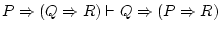Things get harder. Here's the solution to: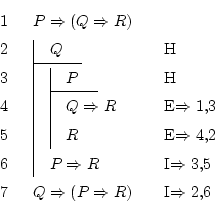But first: here we will only use the two rules that help adding and removing implications, since it's the only operator appearing in the formulas.

We want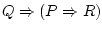, so we will have to do a hypothesis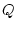inside of which we should prove that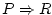. We now do that to simplify the problem: we open a subdemonstration at line 2. We won't close it until we discover thatis true.

Now the problem is somehow easier. We just need to prove, and we have two lines with two truths: the first says that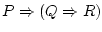, and the second says that.

How can we achieve the? Well, as always: we must suppose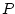, and achieve that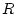is true, in some way. Even if it doesn't seem very simple, it's what must be done, since implication introduction works that way. So we're going to open another hypothesis, now supposing, and let's see if we achieve. This will be a hypothesis inside a hypothesis, but there's no problem in doing that.

After writing line 3, and being inside a subsubdemonstration, we have available that, that, and that. We must prove. Now it isn't that hard, is it? If we know that, we can use implication elimination on line 1, and we will get the true formula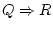. Sinceis also true (line 2), we can apply that rule again to discover that.

We then see that supposingleads us to the conclusion, so we can write down, which is what we wanted. Now we've gone outside the subsubdemonstration, and we're only under the supposition thatis true. As we now see that this supposition implies the truth of the formula, we can end this subdemonstration concluding that.is precisely what had to be proven, so we're finished.

Daniel Clemente Laboreo 2005-05-17